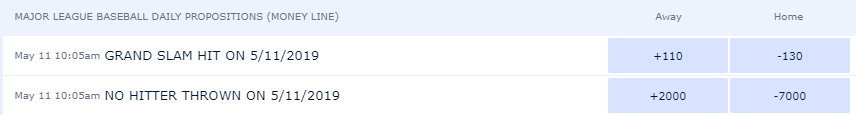## Wizard Recommends

In Final Jeopardy, what is the optimal strategy if the second place player has more than 2/3 the money as the first place player? Assume the third place player is not a factor.

"Anonymous" .

Let me get a disclaimer out of the way first. The following analysis is based on statistical averages. An actual player should make mental adjustments for how well he knows the Final Jeopardy category as well estimating the opponent's chances of getting it correct.

To answer your question, I first looked at four seasons of data from the Jeopardy Archive to see the four possible combinations of the first (leading) and second place (chasing) player getting Final Jeopardy right and wrong.

### Final Jeopardy Scorecard

Leading Player Chasing Player Correct Chasing Player Incorrect Total
Correct 29.0% 25.5% 54.5%
Incorrect 17.7% 27.8% 45.5%
Total 46.8% 53.2% 100.0%

Before going on, let's define some variables:

x = Probability leading player goes high.
y = Probability chasing player goes high.
f(x,y) = Probability of the high player winning.

Let's express f(x,y) in terms of x and y from the table above:

f(x,y) = 0.823xy + 0.545x(1-y) + 0.468(1-x)y + (1-x)(1-y)
f(x,y) = 0.810 xy - 0.455x - 0.532y + 1

To find the optimal values for x and y, let's take the derivative of f(x,y) with respect to both x and y.

f(x,y) d/dx = -0.455 + 0.810y = 0
Thus y = 0.455/0.810 = 0.562

f(x,y) d/dy = -0.532 + 0.810x = 0
Thus x = 0.523/0.810 = 0.657

So, the high player should wager high with probability 65.7% and the low player should wager high with probability 56.2%.

Based on watching, I think the high player wagers high greater than 65.7% of the time, thus if I were in second place, I would go low.

If both players following this randomizing strategy, the probability the leading player will win is 70.1%.

Putting all theory aside, if you're leading, predict what the chasing player will do and do the same. If you're chasing, predict the leading player's action and do the opposite. This strategy goes for all such tournaments.

This question is raised and discussed in my forum at Wizard of Vegas.

I've seen proposition bets at William Hill sports books on whether any Major League Baseball game will have a Grand Slam on a given day. What is the fair probability and line on this by the number of games played?

Sally

For the benefit of other readers, here are the lines for a Grand Slam for May 11, 2019. The column headings should say "yes" and "no," not "home" and "away."To answer this question, I looked at every MLB game from the 2011 to 2018 seasons. In those eight seasons, there were 858 Grand Slams over 19,440 games. That makes the average number of Grand Slams per game 0.0441. This is not the same as the probability any given game will have a Grand Slam, because one game can have more than one. Using the Poisson distribution, the probability of any given game having at least one Grand Slam is 4.3176%.

With that probability it is easy to create the following table showing the probability and fair line for at least one Grand Slam by number of games.

### Grand Slam Probability

Games Probability
Grand Slam
Fair Line
1 4.32% 2216
2 8.45% 1084
3 12.40% 706
4 16.18% 518
5 19.80% 405
6 23.27% 330
7 26.58% 276
8 29.75% 236
9 32.78% 205
10 35.68% 180
11 38.46% 160
12 41.12% 143
13 43.66% 129
14 46.09% 117
15 48.42% 107
16 50.65% -103

I must add a caveat that my answer is based on MLB averages. For any given day, the player should factor in the specific games being played. In particular, the higher the over/under lines, the greater the chances of a Grand Slam.

I found a blackjack machine with a player advantage of 0.1%. As a group, we've played flat bet 2,015,000 hands and am down 1,475 units. I don't specify the exact rules, lest I give the play away, so please take the 0.1% player advantage on faith. What are the odds of running as bad as we are, assuming the game is fair?

"Anonymous" .

Based on that advantage and hands played, the expected win is 2015 units. Assuming a standard deviation of 1.1 per hand, a standard devation on the entire play is 1,561. The difference between your actual win and expected win is 3,490. That is 3,490/1,561 = 2.24 standard deviations. The probability of results that bad or worse is 1.27%.

If I'm betting \$50 on the Ante in Ultimate Texas Hold 'Em I should win \$50 × 500 = \$25,000 on a winning Blind bet. However, the casino caps the win at \$5,000. How much does that cost me on average?

100xOdds

The probability of a winning royal flush is 1 in 32,487. Each time this happens you are shortchanged \$20,000, or 400 Ante bets. That is a cost of 400/32,487 = 1.23% of all money bet on the Ante. That increases the house edge (as measured relative to the Ante bet) from 2.185% to 3.416%.

This question is asked in discussed in my forum at Wizard of Vegas.